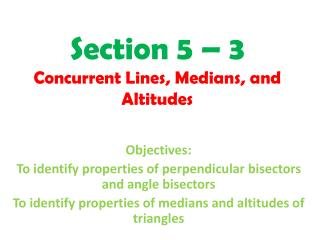DownloadDownload PresentationSection 5 – 3 Concurrent Lines, Medians, and Altitudes

# Section 5 – 3 Concurrent Lines, Medians, and Altitudes

Télécharger la présentation## Section 5 – 3 Concurrent Lines, Medians, and Altitudes

- - - - - - - - - - - - - - - - - - - - - - - - - - - E N D - - - - - - - - - - - - - - - - - - - - - - - - - - -
##### Presentation Transcript

1. Section 5 – 3 Concurrent Lines, Medians, and Altitudes Objectives: To identify properties of perpendicular bisectors and angle bisectors To identify properties of medians and altitudes of triangles

2. Concurrent: When three or more lines intersect in one point. Point of Concurrency: The point at which concurrent lines intersect.

3. Theorem 5 - 6 The perpendicular bisector of the sides of a triangle are concurrent at a point equidistant from the vertices. Circumcenter of the Triangle: The point of concurrency of the perpendicular bisectors of a triangle.

4. Theorem 5 - 7 The bisectors of the angles of a triangle are concurrent at a point equidistant from the sides. Incenter of the Triangle: The point of concurrency of the angle bisectors of a triangle.

5. Point Q, R, and S are equidistant from the circumcenter, so the circle is circumscribed about the triangle. Point X, Y, and Z are equidistant from the incenter, so the circle is inscribed in the triangle.

6. Example 1 Finding the Circumcenter A) Find the center of the circle that you can circumscribe about ∆OPS.

7. B) Find the center of the circle that you can circumscribe about the triangle with vertices (0,0), (-8, 0), and (0, 6).

8. C) Find the center of the circle that circumscribes ∆XYZ.

9. Example 2 Real-World Connection A) The Jacksons want to install the largest possible circular pool in their triangular backyard. Where would the largest possible pool be located?

10. Textbook Page 259 – 260; #1 – 9 (USE GRAPH PAPER)

11. Median of a Triangle: A segment whose endpoints are a vertex and the midpoint of the opposite side

12. Theorem 5 - 8 The medians of a triangle are concurrent at a point that is two thirds the distance from each vertex to the midpoint of the opposite side. Centroid of the Triangle: The point of concurrency of the medians.

13. Example 3 Finding Lengths of Medians A) D is the centroid of ∆ABC and DE = 6. Find BD. Then find BE.

14. B) M is the centroid of ∆WOR, and WM = 16. Find WX.

15. Altitude of a Triangle: The perpendicular segment from a vertex to the line containing the opposite side.

16. Theorem 5 - 9 The lines that contain the altitudes of a triangle are concurrent. Orthocenter of the Triangle: The point of concurrency of the altitudes.

17. Textbook Page 260; # 11 – 22